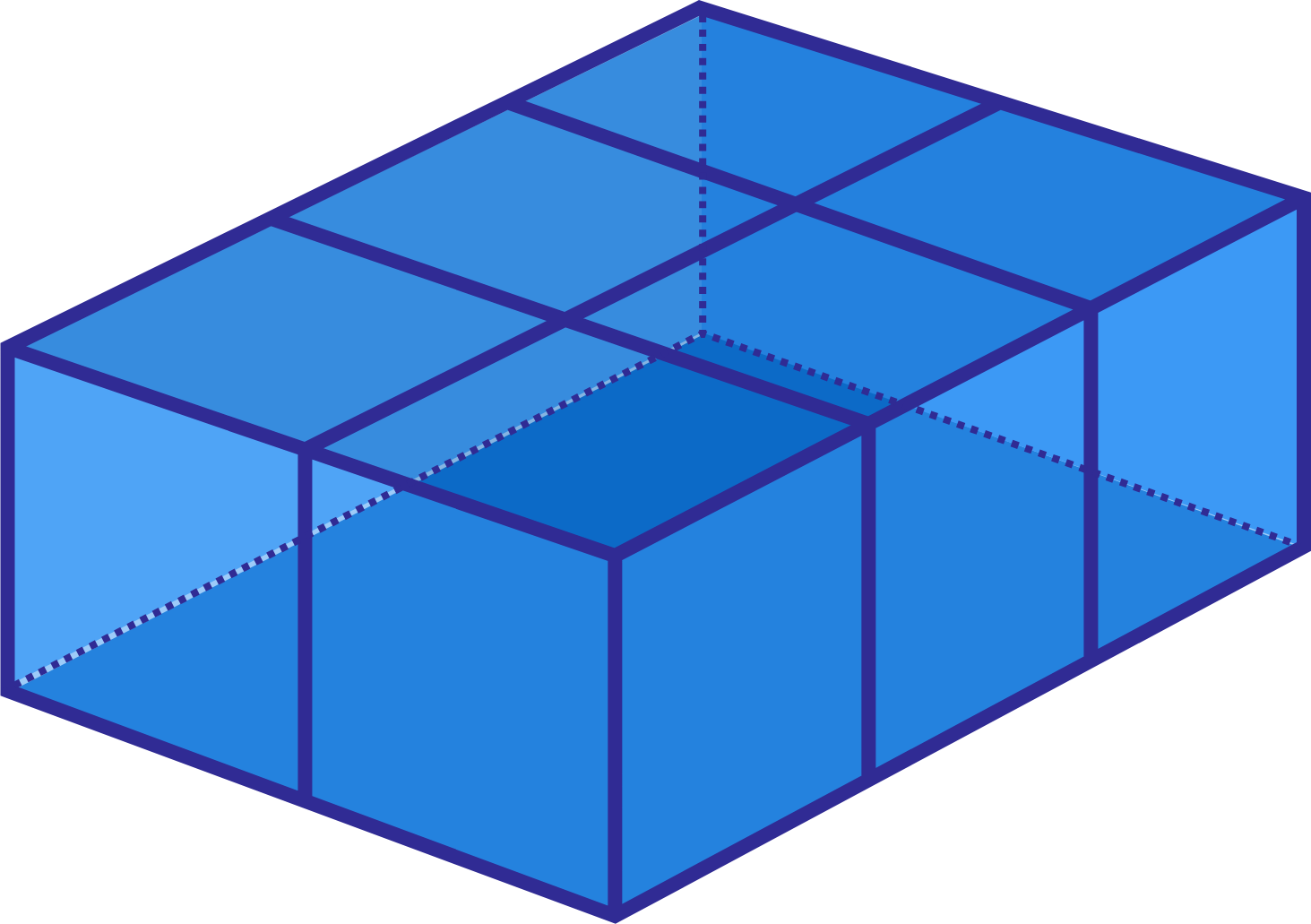# Cuboid cut into cubes

Geometry Level 1If a cuboid of dimensions $10 \text{ cm} × 15 \text{ cm}× 5 \text{ cm}$ is cut to form cubes of sides 5 cm, then what is the difference between the sum of the surface areas of these cubes and the surface area of the original cuboid?

×